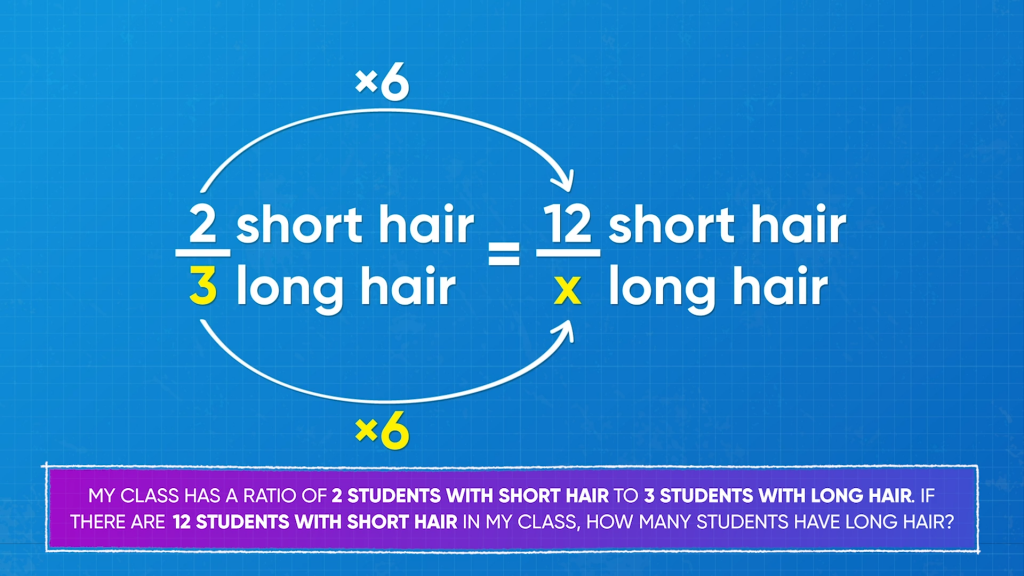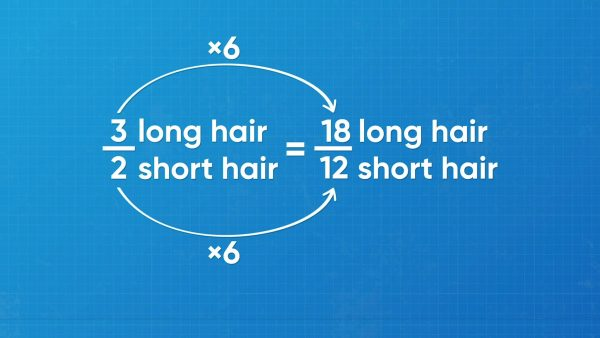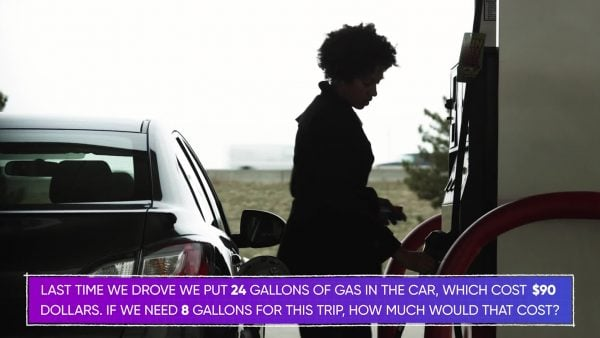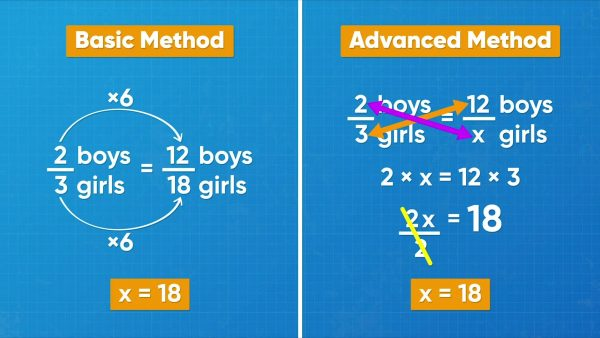Read About Ratios and Proportions | Math for Kids | Grades 6-8
1%
It was processed successfully!WHAT IS SOLVING PROBLEMS WITH RATIOS AND PROPORTIONS?

A ratio tells you the relationship between two parts (part-to-part ratios) or the relationship between a part and a whole (part-to-whole ratios). You can use skills that you already have to solve problems to find quantities using both types of ratios. You can transform word problems into equations to find a more efficient way of solving these problems.

To better understand solving problems with ratios and proportions…

WHAT IS SOLVING PROBLEMS WITH RATIOS AND PROPORTIONS?. A ratio tells you the relationship between two parts (part-to-part ratios) or the relationship between a part and a whole (part-to-whole ratios). You can use skills that you already have to solve problems to find quantities using both types of ratios. You can transform word problems into equations to find a more efficient way of solving these problems. To better understand solving problems with ratios and proportions…

## LET’S BREAK IT DOWN!

### You can use ratios to find unknown quantities.Your class has a ratio of 2 short-haired students to 3 long-haired students. If there are 12 students with short hair in your class, how many students have long hair? You can use a proportion to solve this problem by comparing two ratios. One ratio is 2 short hair to 3 long hair, or 2:3. The other ratio is 12 short hair to an unknown number of long hair, or 12:x. Since the ratios are equivalent, you can set them equal to each other, so 2:3 = 12:x. You need to figure out what numbers to multiply both 2 and 3 by to get the other ratio. 2 × 6 = 12, so multiply 3 by 6 as well. The other ratio is 12:18. There are 18 students with long hair! Now you try: Find x if 3:5 = x:20.

You can use ratios to find unknown quantities. Your class has a ratio of 2 short-haired students to 3 long-haired students. If there are 12 students with short hair in your class, how many students have long hair? You can use a proportion to solve this problem by comparing two ratios. One ratio is 2 short hair to 3 long hair, or 2:3. The other ratio is 12 short hair to an unknown number of long hair, or 12:x. Since the ratios are equivalent, you can set them equal to each other, so 2:3 = 12:x. You need to figure out what numbers to multiply both 2 and 3 by to get the other ratio. 2 × 6 = 12, so multiply 3 by 6 as well. The other ratio is 12:18. There are 18 students with long hair! Now you try: Find x if 3:5 = x:20.

### You can find a smaller quantity from a greater quantity.On a road trip, 24 gallons of gas cost \$90. The next trip requires only 8 gallons of gas. If the price of gas has not changed, how much does the gas cost for the next trip? You can solve this problem using ratios that compare gallons to dollars. The price of gas hasn't changed, so the ratios are equivalent. 24:\$90 = 8:\$x. 24 ÷ 3 = 8, so divide 90 by 3 to make an equivalent ratio. 90÷3=30. 24:\$90 = 8:\$30. 8 gallons of gas cost \$30. Now you try. Find x if 42:77 = x:11.

You can find a smaller quantity from a greater quantity. On a road trip, 24 gallons of gas cost \$90. The next trip requires only 8 gallons of gas. If the price of gas has not changed, how much does the gas cost for the next trip? You can solve this problem using ratios that compare gallons to dollars. The price of gas hasn't changed, so the ratios are equivalent. 24:\$90 = 8:\$x. 24 ÷ 3 = 8, so divide 90 by 3 to make an equivalent ratio. 90÷3=30. 24:\$90 = 8:\$30. 8 gallons of gas cost \$30. Now you try. Find x if 42:77 = x:11.

### You can use ratios with parts to find totals.An animal shelter has a ratio of 4 cats to 5 dogs at their location. If there are 8 cats, how many total animals are there? Read carefully and notice that the ratio compares cats to dogs. You need a ratio that compares cats to total animals. Change the first ratio so that it compares the number of cats to the number of total animals. 4 cats + 5 dogs = 9 total animals. The ratio of cats to total animals is 4:9. We can write a proportion: [ggfrac]4/9[/ggfrac]=[ggfrac]8/x[/ggfrac]. Since we multiply 4 by 2 to get 8, we must also multiply 9 by 2, so we get 18. Then the total number of animals is 18. Now you try: For a special drink recipe, the ratio of lemonade to iced tea is 2:5. If there are 6 cups of lemonade, how many cups of the drink do you have in total?

You can use ratios with parts to find totals. An animal shelter has a ratio of 4 cats to 5 dogs at their location. If there are 8 cats, how many total animals are there? Read carefully and notice that the ratio compares cats to dogs. You need a ratio that compares cats to total animals. Change the first ratio so that it compares the number of cats to the number of total animals. 4 cats + 5 dogs = 9 total animals. The ratio of cats to total animals is 4:9. We can write a proportion: [ggfrac]4/9[/ggfrac]=[ggfrac]8/x[/ggfrac]. Since we multiply 4 by 2 to get 8, we must also multiply 9 by 2, so we get 18. Then the total number of animals is 18. Now you try: For a special drink recipe, the ratio of lemonade to iced tea is 2:5. If there are 6 cups of lemonade, how many cups of the drink do you have in total?

### Use can use ratios with totals to find parts.A salad for a party has 60 cheese balls and cherry tomatoes in all. There are 20 cherry tomatoes. The rest is cheese balls. The tomato to total items ratio is 20:60. Now you want to use the same recipe to make a salad for a bigger party, using 40 tomatoes. How many cheese balls do you need? To set up the ratio, make sure you are consistent with what you are comparing. Change the first ratio to compare tomatoes to cheese balls, just like the second ratio. There are 60 – 20 = 40 cheese balls. The tomato to cheese ratio is 20:40. Since 20 × 2 = 40, multiply 40 × 2 to get 80. You need 80 cheese balls to make the new salad. Now you try: In a pot of chili with a total of 150 beans, 90 are black beans. The rest are pinto beans. You take a bowl of chili and count 30 black beans. How many pinto beans are in your bowl?

Use can use ratios with totals to find parts. A salad for a party has 60 cheese balls and cherry tomatoes in all. There are 20 cherry tomatoes. The rest is cheese balls. The tomato to total items ratio is 20:60. Now you want to use the same recipe to make a salad for a bigger party, using 40 tomatoes. How many cheese balls do you need? To set up the ratio, make sure you are consistent with what you are comparing. Change the first ratio to compare tomatoes to cheese balls, just like the second ratio. There are 60 – 20 = 40 cheese balls. The tomato to cheese ratio is 20:40. Since 20 × 2 = 40, multiply 40 × 2 to get 80. You need 80 cheese balls to make the new salad. Now you try: In a pot of chili with a total of 150 beans, 90 are black beans. The rest are pinto beans. You take a bowl of chili and count 30 black beans. How many pinto beans are in your bowl?

### You can cross-multiply to solve ratios.A more efficient way to solve equivalent ratios is by cross-multiplying. Consider the short-haired versus long-haired problem above. You can write the ratios as fractions instead. 2:3 can be written as 2/3. 12:x can be written as [ggfrac]12/x[/ggfrac]. Set up an equation using the fractions: [ggfrac]2/3[/ggfrac]=[ggfrac]12/x[/ggfrac]. To cross multiply, take the number on the bottom of each fraction and multiply it by the top number on the opposite side to make a new equation. x∙2=12∙3. Now, solve for x. 2x = 36. x=18. As in the solution above, you found that there are 18 long-haired students. Now you try: Use cross-multiplication to solve [ggfrac]4/5[/ggfrac]=[ggfrac]x/20[/ggfrac].

You can cross-multiply to solve ratios. A more efficient way to solve equivalent ratios is by cross-multiplying. Consider the short-haired versus long-haired problem above. You can write the ratios as fractions instead. 2:3 can be written as 2/3. 12:x can be written as [ggfrac]12/x[/ggfrac]. Set up an equation using the fractions: [ggfrac]2/3[/ggfrac]=[ggfrac]12/x[/ggfrac]. To cross multiply, take the number on the bottom of each fraction and multiply it by the top number on the opposite side to make a new equation. x∙2=12∙3. Now, solve for x. 2x = 36. x=18. As in the solution above, you found that there are 18 long-haired students. Now you try: Use cross-multiplication to solve [ggfrac]4/5[/ggfrac]=[ggfrac]x/20[/ggfrac].

## SOLVE PROBLEMS WITH RATIOS AND PROPORTIONS VOCABULARY

Proportion
A comparison of two ratios.
Proportional
Related in the same way through multiplication or division.
Ratio
A comparison between two quantities that can be represented using a fraction, a colon symbol, or words.
Equivalent ratios
Ratios that make the same comparison of numbers.
Cross-multiply
A faster way to solve equivalent ratios of fractions, which comes from multiplying both sides by both denominators.
Part-to-part ratio
A ratio that compares the different parts that make up a total amount.
A ratio that compares one part of an amount to the whole amount.

## SOLVE PROBLEMS WITH RATIOS AND PROPORTIONS DISCUSSION QUESTIONS

### How do you know if the ratios 2:3 and 6:9 are equivalent?

If two ratios are equivalent, that means that I can get one ratio by multiplying or dividing both parts of the other ratio by the same number. Because 2 times 3 is 6, and 3 times 3 is 9, I know that the ratios are equivalent, since I multiplied both parts by 3.

### The ratio of grade 8 students to grade 9 students in a mixed class is 5:3. If there are 10 grade 8 students, how many grade 9 students are there? How can you set up a ratio?

Write the ratio equation 5:3 = 10:x, where x represents the number of grade 9 students. These ratios are proportional. Since I multiply 5 by 2 to get 10, I must also multiply 3 by 2 to get the unknown value. 3 × 2 = 6, so there are 6 grade 9 students.

### You are writing out a recipe that uses 2 tablespoons of brown sugar to 3 tablespoons of white sugar. Should the ratio be 2:3 or 3:2?

I can write the ratio of brown to white as 2:3, or I can write the ratio of white to brown as 3:2. Both are correct, but I have to use the correct ratio when I want to find an equivalent ratio. Suppose you want to make a bigger recipe and you are using 12 tablespoons of brown sugar. Then your ratio would either be brown to white 2:3 = 12:x OR white to brown 3:2 = [ggfrac] x/12[/ggfrac]. Either brown sugar is always the first number in the ratio, or it is always the second number.

### Are [ggfrac]16/12[/ggfrac] and [ggfrac]12/9[/ggfrac] equivalent ratios?

Yes, they are! There is an extra step that helps me find out. I can reduce both ratios to [ggfrac]4/3[/ggfrac]. Since both ratios are equivalent to [ggfrac]4/3[/ggfrac], then they are also equivalent to each other!

### The ratio of apples to oranges in a box is 3:7. A problem asks you to find the total number of fruits if there are 12 oranges. To solve the problem, Josh writes [ggfrac]3/7[/ggfrac] =[ggfrac]12/x[/ggfrac] . Is he correct?

Josh is not correct! The top numbers compare oranges to oranges, which makes sense, but the bottom numbers compare apples to total fruit. The two ratios do not compare the same things. I can change the first ratio so that it also compares the ratio of oranges to total fruit. Add the parts of the ratio to get 3 + 7 = 10. Then the ratio of oranges to total fruit is [ggfrac]3/10[/ggfrac] . The correct equation can be [ggfrac]3/10[/ggfrac] =[ggfrac]12/x[/ggfrac] , which I can solve to find that there are 40 fruits in all.
X

## Success

We’ve sent you an email with instructions how to reset your password.
Ok